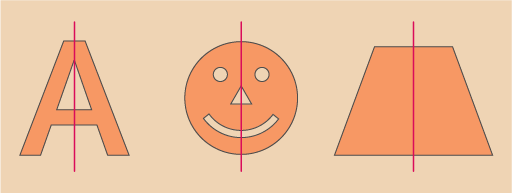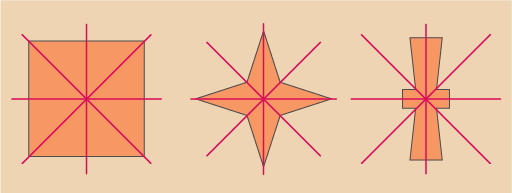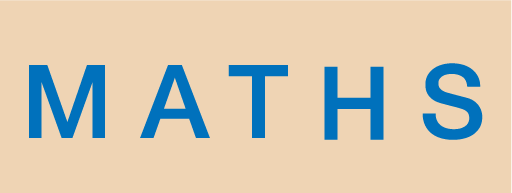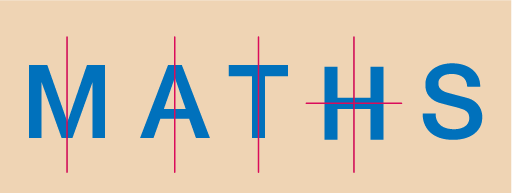Science, Maths & Technology

### Become an OU studentEveryday maths 1 (Wales)

Start this free course now. Just create an account and sign in. Enrol and complete the course for a free statement of participation or digital badge if available.

# 2 Symmetry

A 2D symmetrical shape can be folded in half so that both sides are the same. The fold is called a line (or lines) of symmetry.

The shapes in Figure 10 have one line of symmetry.Figure 10 One line of symmetry

Some shapes, such as the middle one above, will only have one line of symmetry because of the details included, like the eyes, nose and mouth. However, a circle with no added details has an infinite number of lines of symmetry!

The shapes in Figure 11 have multiple lines of symmetry.Figure 11 Multiple lines of symmetry

Now try the following activity.

## Activity 5: Lines of symmetry

How many lines of symmetry in Figure 12 does each of these letters have?Figure 12 How many lines of symmetry?Figure 13 Lines of symmetry

‘M’, ‘A’ and ‘T’ have one line of symmetry.

‘H’ has two lines of symmetry.

‘S’ has no lines of symmetry.

## Activity 6: How many lines of symmetry?

Sometimes a line of symmetry is called a mirror line, because if you placed a mirror along the line the shape would look the same.

Try writing your name in capital letters and seeing how many lines of symmetry each letter has. You could use a mirror to check your answers.